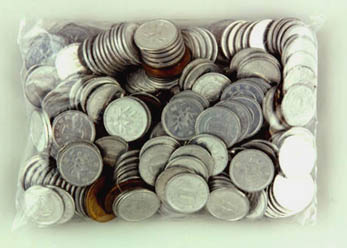to english | index | next

220枚の硬貨から成る284円 (金額第一二番)
284 Yen Which Consists of 220 Coins (Money Amount No. 12)

Japanese coins, a plastic bag
2001
photo by Takagi Shigeki

cf. 220 and 284 are the amicable pair which Pythagoras et al. found in about 500 B. C.
(Two numbers are called amicable if each equals to the sum of the divisors of the other.)
Divisors of 220: 1 + 2 + 4 + 5 + 10 + 11 + 20 + 22 + 44 + 55 + 110 = 284
Divisors of 284: 1 + 2 + 4 + 71 + 142 = 220